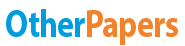# Advance Project Management Study Guide

Essay by   •  July 16, 2019  •  Study Guide  •  1,048 Words (5 Pages)  •  280 Views

## Essay Preview: Advance Project Management Study Guide

Report this essay
Page 1 of 5

Density(D):  – where L= number of activities in network– pi = number of predecessor activities – si= number of successor activities (not including start and finish)[pic 1]

1. Create four (4) additional columns on the sheet provided. Columns should be labeled P, S, P-S & MAX.
2. P is determined by the number of immediate processor activities and S is determined by the number of following activities. P-S is just simple math. MAX of each activity cannot be a negative. If the number is negative, it is given a value of zero (0).
3. After you have all the MAX values, you total those values to get the Density of the project.

1≤D≤5; benchmark ≥2 | 5

 Utilization ():[pic 2] Network Complexity (λ) [pic 3]Create three (3) columns additional columns on the sheet provided. Columns should be labeled W is should be for all of the number of resources required columns. W is calculated by the Duration Time multiplied by the number of reusable resources all totals would then be added for the W total. [pic 4][pic 5]

Determining the project duration without resources

This is based on the network diagram.

Determining the rank of activities

1. Start by drawing the network diagram for the project.
2. Fill in the given chart with the activities, duration, predecessor activities and the resources required.
3. The Activity ROT is equal to the resources required divided by the duration(time) of the activity. (ROT = Resource/Time) [i.e. if the resource is 5 and the duration is 10 the ROT is 5/10=.5]
4. After you have determined the ROT, you now need to complete the sets for each activity. The number of sets for the activities are determined based the number of successor activities (not including finish).
5. After determining the number of sets you will have and
6. Based on the below network diagram, activities E would have two sets while the reminding activities would have one set.

[A set 1=A activity ROT+B activity ROT+C activity ROT | B set 1=B activity ROT+C activity ROT | C set 1=C activity ROT | D set 1=D activity ROT+E activity ROT | E set 1=E activity ROT+C activity ROT & E set 2=E activity ROT|F set 1=F activity ROT+G activity ROT | G set 1=G activity ROT]

[pic 6]

1. After the sets has been determined, you take the max value to for the overall ROT. [i.e. if E sets where equal to 1.5 and 2, the overall ROT would be 2.
2. After the overall ROT has been determined, you will now rank the ROT from highest to lowest. [if there are activities with the same ROT, those activities ranks will be determined by 1. The largest duration, 2. # of predecessor activities, 3. # of successor activities, 4. Amount of resources needed.

Determining the minimum duration allowed with max resources

 Steps 1 ROT This is determined based on the rank of activities (NOTE: Activities must be transferred in order form highest to lowest.) 2 Duration This is determined based on the given duration of the activity. 3 Resources Required This is determined based on the resources needed to complete the activity. 5 Early Start This is determined based on the network diagram and the ES given. (the number on the top left of the box) 9 Time Start This is determined based on when the activity actually starts. Although in some cases the time start will be the same at the early start, but if resources aren't available, than it will be different. 10 Time Finish This is determined based on the Time Start (step 9) + the Duration (step 2) of the activity. 4 Resource Released @ Finish Time This will be the same as the # of resources needed to complete the activity. 6 Time Now This is determined based on the lowest early start value. After your first column has been completed, this is based on Early Start and Time Finished. (this will always start at zero) 7 Resource Available This is determined based on the Total # of Resources available per period and will be given to us. 8 Activity Allowed You now need to go back to your early start row to determine what activities can start at that period. The total resources for activities cannot exceed the resources available. If it does, you have to wait for another period when the resources are available. After resources have been used, you should reflect that (by crossing out the number and replacing it with the new number to reflect the resources that will be available. 11 Iteration # This is determined based on if an activity was allowed to start or not After you have completed the first column, you need to redo steps 6-11 for the remaining time now columns. This time, you need to look at what activities are also finishing at the time period as well to make sure your resources available is correct.

...

...